# Regular extremal

(diff) ← Older revision | Latest revision (diff) | Newer revision → (diff)

non-singular extremal

An extremal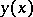at all points of which the following condition holds:(1)

where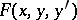is the integrand appearing in a functional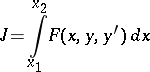which is to be minimized. Like any extremal, a regular extremal is, by definition, a smooth solution of the Euler equation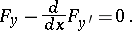The points of an extremal at which (1) holds are called regular points. It is known that at every regular point, an extremalhas a continuous second-order derivative. On a regular extremal, the second-order derivative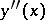is continuous. For a regular extremal the Euler equation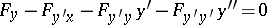can be written in the following form (that is, solved for the highest derivative):The regularity property (1) is directly connected with the necessary Legendre condition (in the strong form), according to which at all points of the extremal the following inequality holds:Essential use is made of regularity when proving that an extremalcan be included in a field of extremals surrounding it. If condition (1) is violated at even one point, the extremal cannot always be included in a field. This condition for including the extremal in a field is one of the sufficient conditions for being an extremal.

The above definition of a regular extremal is given for the simplest problem of the calculus of variations, which concerns functionals depending on one unknown function. For functionals depending onunknown functions,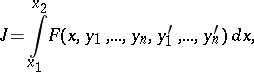a regular extremal is an extremal for which at every point the-th order determinant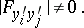(2)

In some general problems of the calculus of variations on a conditional extremum (see Bolza problem), a regular extremal is defined in a similar way, except that in (2) instead ofone must put the Lagrange function.

An extremal for which the regularity condition ((1) or (2)) is violated at every point of some section is called a singular extremal, and the section is called a section of singular regime. For singular regimes there are necessary conditions supplementing the known classical necessary conditions for an extremum (see Optimal singular regime).

How to Cite This Entry:
Regular extremal. Encyclopedia of Mathematics. URL: http://encyclopediaofmath.org/index.php?title=Regular_extremal&oldid=17757
This article was adapted from an original article by I.B. Vapnyarskii (originator), which appeared in Encyclopedia of Mathematics - ISBN 1402006098. See original article